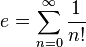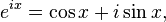e’s are good – He’s Leonard Euler.

Along with pi, e is one of the most important constants in mathematics. It is an irrational number which carries on forever. The first few digits are 2.718281828459045235…

Leonard Euler

e is sometime named after Leonard Euler (Euler’s number).   He wasn’t the first mathematician to discover e – but he was the first mathematician to publish a paper using it.    Euler is not especially well known outside of mathematics, yet he is undoubtedly one of the true great mathematicians.  He published over 800 mathematical papers on everything from calculus to number theory to algebra and geometry.

Why is e so important?

Lots of functions in real life display exponential growth. Exponential growth is used to describe any function of the form ax where a is a constant. One example of exponential growth is the chessboard and rice problem, (if I have one grain of rice on the first square, two on the second, how many will I have on the 64th square?) This famous puzzle demonstrates how rapidly numbers grow with exponential growth.

Sketch
y = 2x
y = ex
y = 3x

for between x = 0 and 3. You can see that y = ex is between y=2x and y = 3x on the graph, so why is e so much more useful than these numbers? By graphical methods you can find the gradient when the graphs cross the y axis. For the function y = ex this gradient is 1. This is because the derivative of ex is still ex – which makes it really useful in calculus.

The beauty of e.

e appears in a host of different and unexpected mathematical contexts, from probability models like the normal distribution, to complex numbers and trigonometry.

Euler’s Identity is frequently voted the most beautiful equation of all time by mathematicians, it links 5 of the most important constants in mathematics together into a single equation.Infinite fraction: e can be represented as a continued infinite fraction can students you spot the pattern? – the LHS is given by 2 then 1,2,1 1,4,1 1,6,1 etc.Infinite sum of factorials: e can also be represented as the infinite sum of factorials:A limit: e can also be derived as the limit to the following function.  It was this limit that Jacob Bernoulli investigated – and he is in fact credited with the first discovery of the constant.Complex numbers and trigonometry :  e can be used to link both trigonometric identities and complex numbers:You can explore more of the mathematics behind the number e here.

If you enjoyed this post you might also like:

Ramanujan’s Beauty in Mathematics Some of the amazingly beautiful equations of Ramanujan.IB RevisionIf you’re already thinking about your coursework then it’s probably also time to start planning some revision, either for the end of Year 12 school exams or Year 13 final exams. There’s a really great website that I would strongly recommend students use – you choose your subject (HL/SL/Studies if your exam is in 2020 or Applications/Analysis if your exam is in 2021), and then have the following resources:The Questionbank takes you to a breakdown of each main subject area (e.g. Algebra, Calculus etc) and each area then has a number of graded questions. What I like about this is that you are given a difficulty rating, as well as a mark scheme and also a worked video tutorial.  Really useful!The Practice Exams section takes you to ready made exams on each topic – again with worked solutions.  This also has some harder exams for those students aiming for 6s and 7s and the Past IB Exams section takes you to full video worked solutions to every question on every past paper – and you can also get a prediction exam for the upcoming year.

I would really recommend everyone making use of this – there is a mixture of a lot of free content as well as premium content so have a look and see what you think.